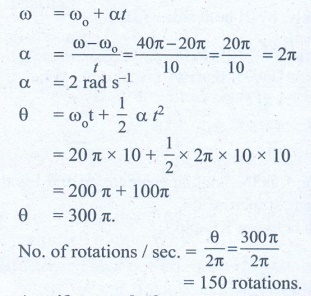Home | | Physics 11th std | Book Back Numerical Problems

# Book Back Numerical Problems

Physics : Motion of System of Particles and Rigid Bodies : Book Back Exercise, Example Numerical Question with Answers, Solution : Book Back Numerical Problems

Motion of System of Particles and Rigid Bodies | Physics

## 1. A uniform disc of mass 100g has a diameter of 10 cm. Calculate the total energy of the disc when rolling along a horizontal table with a velocity of 20 cms-1. (take the surface of table as reference)AnswerMass of the disc m = 100 g = 0.1 kg.Diameter of the disc d = 10 cmRadius of the disc r = 5 cm = 0.05mRolling with a velocity v = 20 cms-1 = 0.20 ms-1Total energy of the disc ETot = ?ETot = Translational K.E. + rotational K.E.Moment of inertia (M.I) of the disc about its own axisAns: 0.1028 J2. A particle of mass 5 units is moving with a uniform speed of v = 3√2 units in the XOY plane along the line y = x + 4. Find the magnitude of angular momentum.AnswerEquation of line x - y + 4 = 0Mass of particle = 5 unitsSpeed v - 3 √2 unitsDistance of line from origin r =Angel momentum L = mvr = 5 × 3 √2 × 4/√2 = 60 unitsAns: 60 units3. A fly wheel rotates with a uniform angular acceleration. If its angular velocity increases from 20π rad/s to 40π rad/s in 10 seconds. Find the number of rotations in that period.Answer:Initial Angular Velocity ω0 = 20π rad s-1Final Angular velocity ω = 40 π rad s-1time taken, t  = 10 sNo. of rotations / s = ?= 150 rotations.Ans: 150 rotations4. A uniform rod of mass m and length l makes a constant angle θ with an axis of rotation which passes through one end of the rod. Find the moment of inertia about this axis.Answerθ - angle that rod makes with the axis (vertical)Linear mass density λ = m/lConsider dx. the part of rodMass dx, the part of rod dm=λ.dxdm= m/l dx. The M.I of the rod about OO'I = ∫ r2 dmWhere is the perpendicular distance from the elementary mass dm.M.I about the axis OO' = 1/12 ml2.sin2 θ.Ans: 1/12  Ml 2 sin2 θ5. Two particles P and Q of mass 1kg and 3 kg respectively start moving towards each other from rest under mutual attraction. What is the velocity of their center of mass?AnswerMass of particle P m1 = 1 kg.Mass of particle Q m2 = 3 kgVelocity of particle P = velocity of particle Qbut in opposite direction.velocity of center of mass vcm = ?Ans: Zero6. Find the moment of inertia of a hydrogen molecule about an axis passing through its center of mass and perpendicular to the inter-atomic axis. Given: mass of hydrogen atom 1.7 × 10-27 kg and inter atomic distance is equal to 4 × 10-10 m.Ans: 1.36 × 10-46 kg m27. On the edge of a wall, we build a brick tower that only holds because of the bricks’ own weight. Our goal is to build a stable tower whose overhang d is greater than the length l of a single brick. What is the minimum number of bricks you need?(Hint: Find the center of mass for each brick and add.)8. The 747 boing plane is landing at a speed of 70m s-1. Before touching the ground, the wheels are not rotating. How long a skid mark do the wing wheels leave (assume their mass is 100 kg which is distributed uniformly, radius is 0.7 m, and the coefficient of friction with the ground is 0.5)?Ans: 2.1 m

Tags : Motion of System of Particles and Rigid Bodies | Physics , 11th Physics : UNIT 5 : Motion of System of Particles and Rigid Bodies
Study Material, Lecturing Notes, Assignment, Reference, Wiki description explanation, brief detail
11th Physics : UNIT 5 : Motion of System of Particles and Rigid Bodies : Book Back Numerical Problems | Motion of System of Particles and Rigid Bodies | Physics